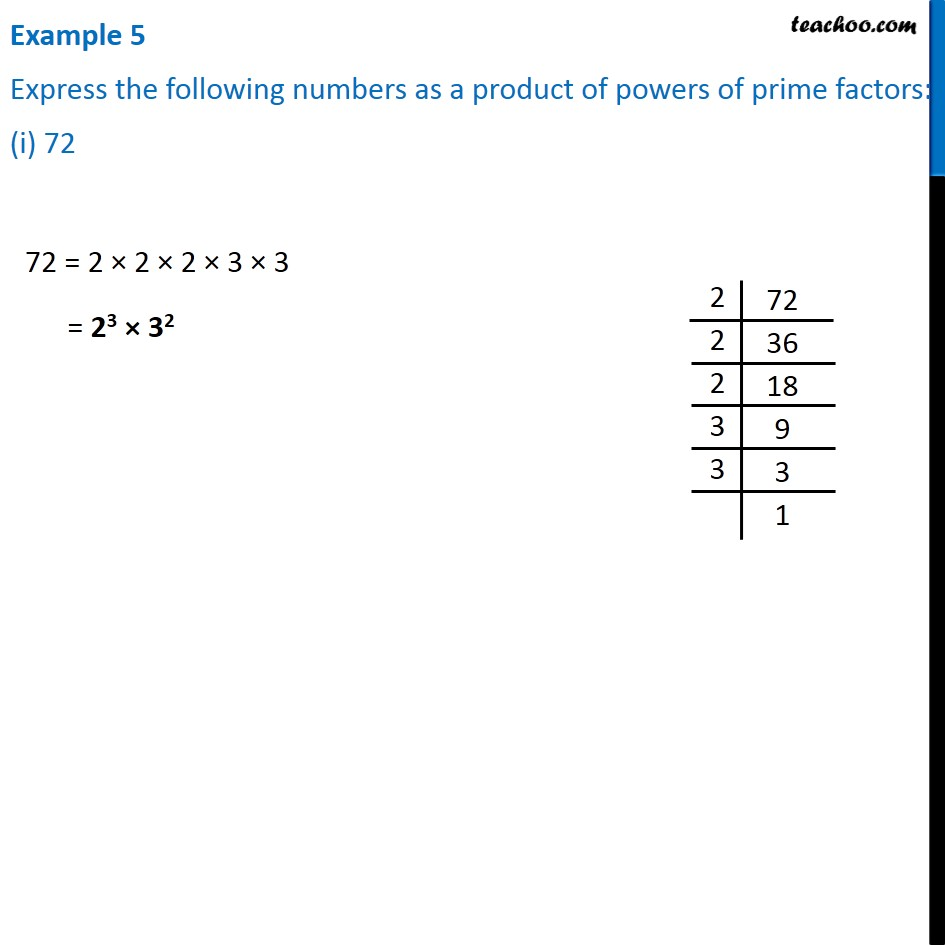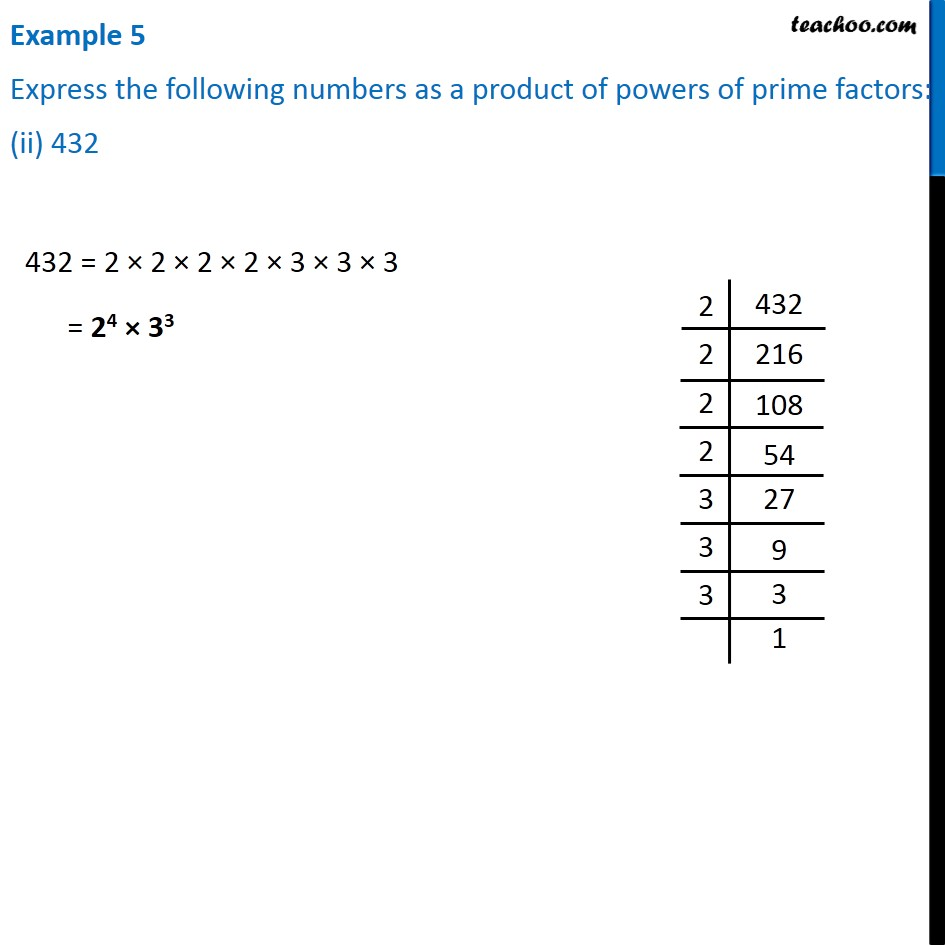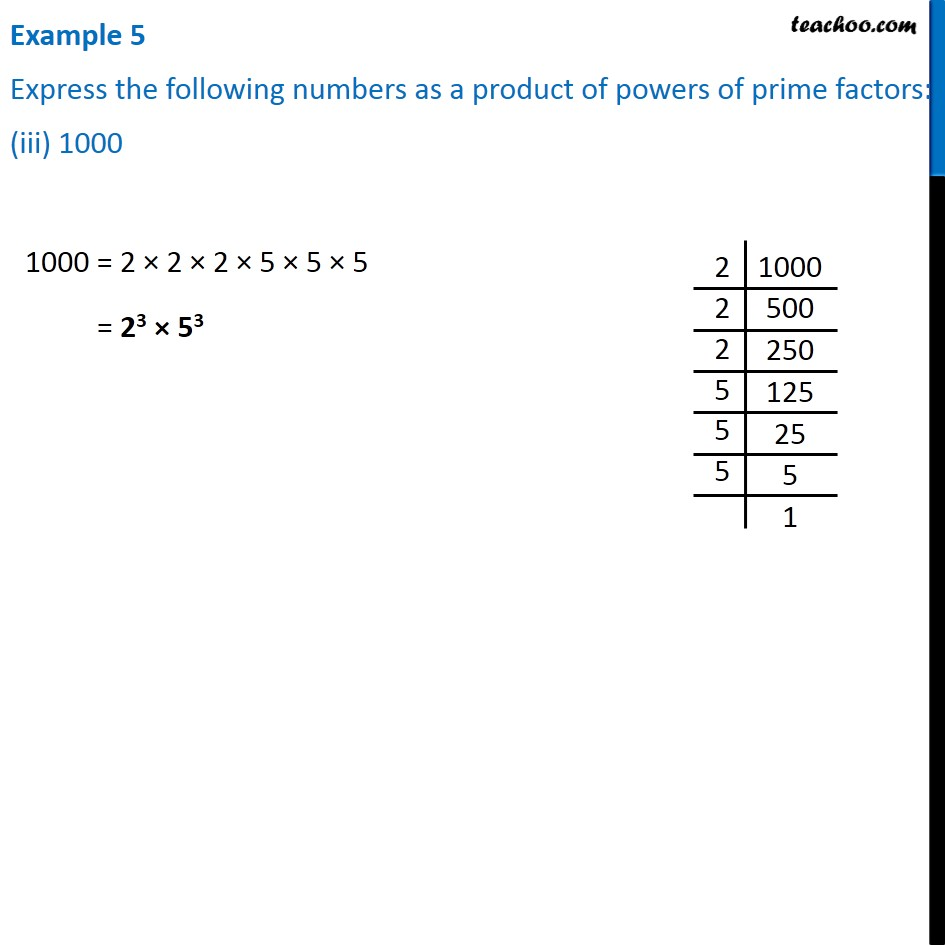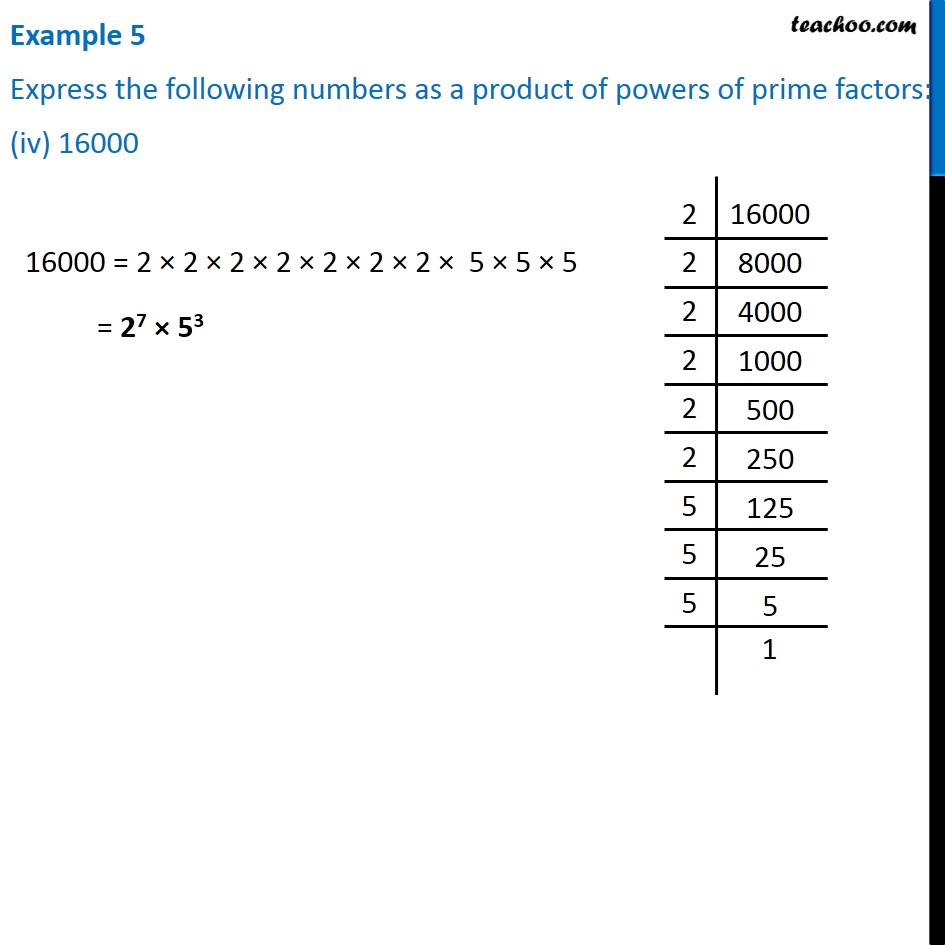1. Chapter 13 Class 7 Exponents and Powers
2. Serial order wise
3. Examples

Transcript

Example 5 Express the following numbers as a product of powers of prime factors: (i) 72 72 = 2 × 2 × 2 × 3 × 3 = 23 × 32 2|72 2|36 2|18 3|9 3|3 |1 Example 5 Express the following numbers as a product of powers of prime factors: (ii) 432 432 = 2 × 2 × 2 × 2 × 3 × 3 × 3 = 24 × 33 2|432 2|216 2|108 2|54 2|27 3|9 3|3 |1 Example 5 Express the following numbers as a product of powers of prime factors: (iii) 1000 1000 = 2 × 2 × 2 × 5 × 5 × 5 = 23 × 53 2|1000 2|500 2|250 5|125 5|25 5|5 |1 Example 5 Express the following numbers as a product of powers of prime factors: (iv) 16000 16000 = 2 × 2 × 2 × 2 × 2 × 2 × 2 × 5 × 5 × 5 = 27 × 53 2|16000 2|8000 2|4000 2|1000 2|500 2|250 2|125 2|25 5|5 1|

Examples